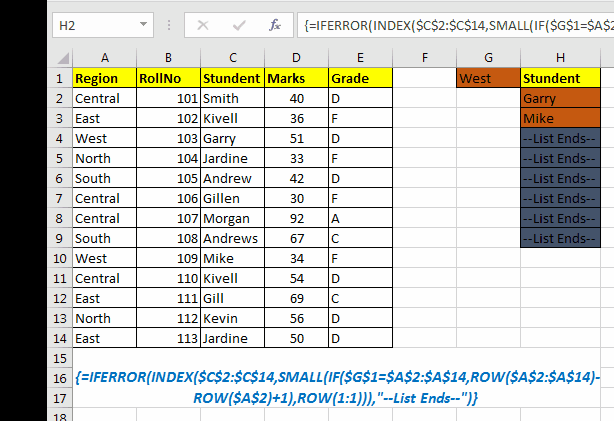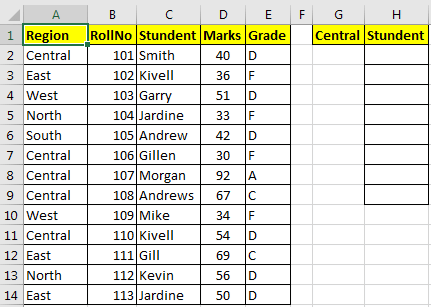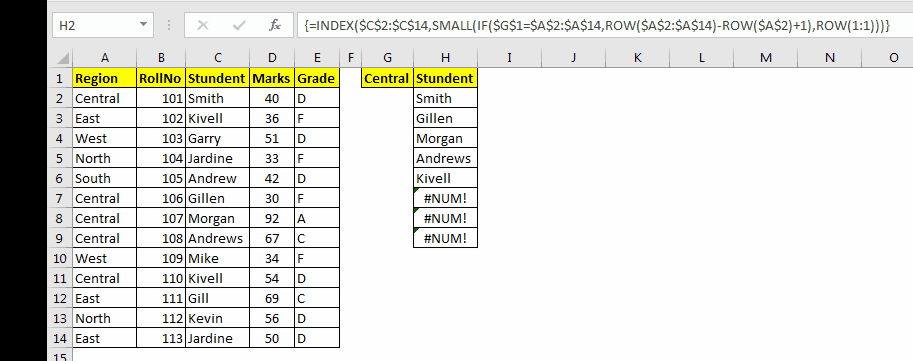# How to LOOKUP Multiple Values

If you are here, it means that you are trying to use VLOOKUP to retrieve multiple values from a data set. But let’s get it straight, VLOOKUP CAN’T RETURN MULTIPLE VALUES. But that doesn’t mean we can’t do it. We can do a lookup that retrieves multiple values instead of the first one only.Generic Formula

{=INDEX(array,SMALL(IF(lookup_value=lookup_value_range,ROW(lookup_value_range)-ROW(first cell of lookup_value_range)+1),ROW(1:1)))}

Array: The range from where you want to fetch data.
lookup_value: Your lookup_value that you want to filter.
lookup_value_range: The range in which you want to filter lookup_value.
The first cell in lookup_value range: if your lookup_value range is \$A\$5:\$A\$100 then its \$A\$5.
Important: Everything should be absolute referenced. lookup_value can be relative according to requirement.
Enter it as an array formula. After writing formula hit CTRL+SHIFT+ENTER key to make it an array formula.

### Example of lookup for multiple results

I have this student data in range A2:E14. In cell G1, I have a drop-down of region values e.g. Central, East, North, South, and West. Now I want, whichever region I have in G1, a list of all students from that region should be displayed in the H column.To lookup multiple values in excel, let’s identify our variables.
Array: \$C\$2:\$C\$14
lookup_value: \$G\$1
lookup_value_range: \$A\$2:\$A\$14
The first cell of lookup_value range: \$A\$2
According to the above data, our formula to retrieve multiple values in excel will be:

{=INDEX(\$C\$2:\$C\$14,SMALL(IF(\$G\$1=\$A\$2:\$A\$14,ROW(\$A\$2:\$A\$14)-ROW(\$A\$2)+1),ROW(1:1)))}

Copy this formula in H2, and hit CTRL+SHIFT+ENTER. Now drag down this formula until you get a #NUM error. #NUM indicates that there are no other remaining matching values to that lookup_value, and it is the end of the list.If #NUM annoys you, you can have the IFERROR function in front of the formula and display some meaningful info instead of the error.

{=IFERROR(INDEX(\$C\$2:\$C\$14,SMALL(IF(\$G\$1=\$A\$2:\$A\$14,ROW(\$A\$2:\$A\$14)-ROW(\$A\$2)+1),ROW(1:1))),"--List Ends--")}

This will show --List Ends-- when all items are shown.

### Now let’s understand HOW IT WORKS.

Even though the formula may look complex, but the idea is simple. We need to get the index number of each occurrence of value, then retrieve values using the INDEX function of Excel.

Hence the main challenge is of getting an array of index numbers of lookup_value. To get index numbers, we used IF and ROW functions. The formula is indeed complex altogether, let’s break it down.

We want to get values from the student column, so our array for INDEX function is \$C\$2:\$C\$14.

Now we need to give row numbers from \$A\$2:\$A\$14 (lookup value) in which G1’s value exists (for now, let’s say G1 has central in it).

IF(\$G\$1=\$A\$2:\$A\$14,ROW(\$A\$2:\$A\$14): Now, this part returns row number if a cell countain’s value of G1 (central) in range \$A\$2:\$A\$14 else returns FALSE. In this example, it’ll return

{2;FALSE;FALSE;FALSE;FALSE;7;8;FALSE;FALSE;11;FALSE;FALSE;FALSE}.

• Now, since the above array contains row number from 1st row (1:1) and we need row starting from our array (A2:A14). To do so, we use -ROW(\$A\$2)+1 in IF formula. This will return a number of row before starting of our array.

For this example, it is -1. If it was starting from A3, it would return -2 and so on. This number will be subtracted from each number in the array returned by IF. So Finally IF(\$G\$1=\$A\$2:\$A\$14,ROW(\$A\$2:\$A\$14)-ROW(\$A\$2)+1) this will translate to {1;FALSE;FALSE;FALSE;FALSE;6;7;FALSE;FALSE;10;FALSE;FALSE;FALSE}.

• Next, this array is surrounded by SMALL function. This function returns the Nth smallest value in the given array. Now, we have SMALL({2;FALSE;FALSE;FALSE;FALSE;7;8;FALSE;FALSE;11;FALSE;FALSE;FALSE},ROW(1:1)). ROW(1:1) will return 1. Hence, the above function will return 1st smallest value in the array, which is 2.

When you’ll copy this formula in the below cells ROW(1:1) will become ROW(2:2) and it will return the 2nd smallest value in the array, which is 7 and so on. This allows the function to return first found value first. But if you want to get last found value first then use the LARGE Function instead of SMALL function.

Now using values returned above functions, the INDEX function easily returns each matching value from a range.

So, yeah guys you can LOOKUP multiple corresponding values by one lookup value. In the gif above, I have used the IFERROR function to catch errors and conditional formatting to make it a little bit visual. In the above example, I have used many other functions and techniques that I have explained in other articles.

Related Articles:

How to Use Excel INDEX Function

How to Use The VLOOKUP Function in Excel

How to Use Excel SMALL function

How to Use The Excel IF Function

How to Lookup Value with Multiple Criteria

How to Lookup Top 5 Values with Duplicate Values Using INDEX-MATCH in Excel

How to Use VLOOKUP with numbers and text

## Users are saying about us...

1.Best lesson

•Thanks!

2.This was very helpful! Is it possible to filter on the values that are returned in column H? I am not being able to filter those. Only if I filter on the original data in column C.

3.its not working

Terms and Conditions of use

The applications/code on this site are distributed as is and without warranties or liability. In no event shall the owner of the copyrights, or the authors of the applications/code be liable for any loss of profit, any problems or any damage resulting from the use or evaluation of the applications/code.